# Research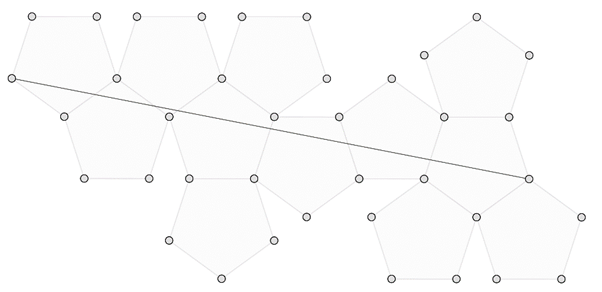Platonic solid – Dodecahedron

### David Aulicino

#### Geometry and Dynamical Systems

My research involves the patterns made by a single billiard ball on a polygonal table. It relates to geometry and dynamical systems. However, it touches on many other fields. Recently, I studied the Platonic solids and proved a new theorem about the dodecahedron. I am happy to work with any student on an Independent Study course if they have satisfied the requirements of the department. If you are interested in taking MATH 5001 with me, contact me a semester beforehand so that we can discuss your interests and find a common ground for study! My research is supported by the National Science Foundation and PSC-CUNY.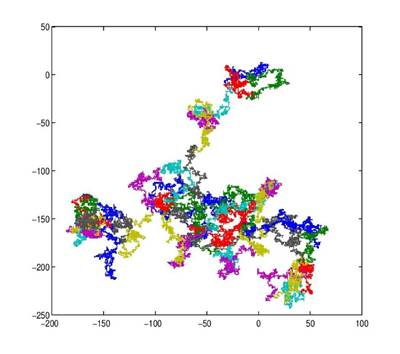Random Fractal Processes

### Christian G. Benes

#### Probability and Statistical Mechanics

I study the fundamental properties of random fractal processes such as simple random walk, loop-erased random walk, Brownian motion, and the Schramm-Loewner Evolution. A simple random walk is a random process where a particle (the “walker”) moves at each time step in one of several directions with equal probability. While this process has been around for a long time and has been used extensively as a model for phenomena from physics, chemistry, biology, as well as for the stock market, there are still plenty of exciting questions about it that haven’t been answered. I can supervise independent studies for students who are interested in probability, analysis, and financial or actuarial mathematics.

### Reza Chamanara

#### Low Dimensional Geometry and Topology

My research revolves around study of geometric structures on surfaces and three-dimensional manifolds. My work mixes methods from various parts of mathematics including hyperbolic geometry, low dimensional topology, combinatorial geometry, complex analysis, and the algebraic study of discrete matrix groups. I have long been interested in certain geometric invariants associated with simple closed curves in the plane which are defined by the convex hull of these curves inside the three-dimensional hyperbolic space. As one of the ingredients of this project I have also studied various patterns of geometric disks on surfaces. Some of these disk patterns have also been studied in the context of creating a combinatorial theory of conformal maps on surfaces and my hope is that part of my work will be applicable in that area. If you have the needed requirements and subject to finding a proper time that works for me as well as for you, I should be able to advise you for any independent study course or undergraduate research project within the mathematical fields close to my interest.

### Anthony E. Clement

#### Combinatorial Group Theory—One Relator and Nilpotent Groups

My research is in infinite group theory. My primary focus is in Combinatorial Group Theory, in the study of one relator groups and lately in nilpotent groups. In short, Combinatorial Group Theory is the study of groups via generators and relations. There are three main problems that drives research in Combinatorial Group Theory: The Word Problem, The Conjugacy Problem, and the Isomorphism Problem. The hardest of the three problems is the Isomorphism Problem. My research involves finding solutions for the Isomorphism Problem in certain groups. I can supervise independent study projects for students who have taken Abstract Algebra I and Abstract Algebra II.

### Laurel Cooley

#### Sociology of Mathematics Education and Cognition of College Mathematics

My current research examines issues of race and equity in mathematics education, particularly in urban schools. This research was generated from a project I directed as a part of the MetroMath—Mathematics in America’s Cities initiative. Building on this, I am currently leading a research study examining the career trajectories of selective alternative certification mathematics teachers, and connected issues of race, equity, and opportunity in mathematics education. I am also currently serving as a researcher and evaluator on a project to reform mathematics departments to better incorporate assessments of proof based on mathematics education research. I have also published extensively on research about the development of understanding of undergraduate mathematics, e.g. calculus and linear algebra concepts. My research is supported by the National Science Foundation. I can supervise independent study projects for students who are interested in equity issues in mathematics education or cognition of college mathematics.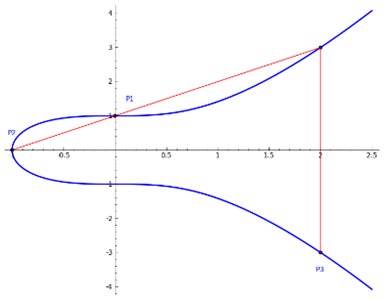Algebraic Curves

### Heidi Goodson

#### Number Theory and Algebraic Curves

My research in the field of number theory focuses on studying arithmetic properties of curves and higher dimensional varieties over finite fields. In particular, number theorists like myself are most interested in studying elliptic curves. Questions of how to find, count, and characterize points on elliptic curves have been studied since the days of Diophantus. I am currently studying how the number of points on these and other curves varies as the order of the finite field varies. This area of number theory is commonly known as the study of Sato-Tate Distributions. Furthermore, I am also investigating the potential for elliptic curves to be used in post-quantum cryptography. I can supervise independent study projects for students who have taken at least one semester of Abstract Algebra.

### Noemi Halpern

#### Riemann Surfaces, Kleinian Groups, and Mathematics Education

My primary areas of mathematical interest are Riemann surfaces and Kleinian groups. I have studied moduli of Riemann surfaces and Nielsen extensions. I have also done work in math education for the general population, and in particular for the learning-disabled population. I enjoy working with students interested in a broad range of areas—real and complex analysis, algebra, number theory, and math education.

### Jun Hu

#### Low-Dimensional Dynamical Systems and Teichmüller Spaces

My first main research interest lies in the study of mathematical definitions of chaos, bifurcations, and transitions from non-chaotic to chaotic systems. In this area, I also work with fractal sets, such as Julia sets of rational maps and Mandelbrot sets in the parameter plane, and study ergodicity of smooth one-dimensional dynamical systems, which relates to inseparability of a system on a chaotic region. My second major interest lies in the study of geometric structures on so-called Teichmüller spaces, which introduces metrics and Banach manifold structures on sets of different hyperbolic or conformal structures on Riemann surfaces other than the flat plane. I can supervise students who have taken MATH 2206 (Ordinary Differential Equations) and MATH 4201 (Advanced Calculus I), or MATH 2706 (Dynamical Systems) and MATH 4216 (Functions of a Complex Variable).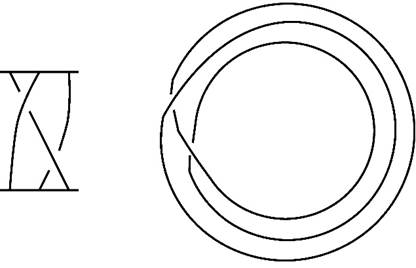Knot / Braid Theory

### Diana Hubbard

#### Knot Theory, Low-Dimensional Topology, and Braid Theory

My research primarily involves studying knots and three-dimensional manifolds by drawing on techniques from braid theory. A mathematical knot is constructed as follows: take a piece of string, tie it up in some way, and then glue the ends together. The beauty of knot theory is that even though knots are easy to visualize, it is often surprisingly hard to answer even very simple questions about them. Knot theory has applications not only to many other areas of mathematics but also to physics, biology, and chemistry. I can supervise independent study projects for students who are familiar with advanced calculus and abstract algebra. My research is partially supported by the American Mathematical Society and Simons Foundation.

### Sandra Kingan

#### Matroid Theory, Graph Theory, and Network Science

My specialty, Matroid Theory, captures the notion of independence across several areas of mathematics including projective and affine spaces, lattice theory, graph theory, error correcting codes, and combinatorial optimization. Matroids are a modern type of geometry where the behavior of points, lines, planes, and higher dimensional surfaces is governed by combinatorial axioms. Binary matroids are matroids that can be represented by binary matrices and graphs (also called networks) are a subclass of binary matroids. In the past few decades the ability to analyze large graphs with computers has resulted in a new area called Complex Networks. Examples of complex networks include the neurons in the brain linked by synapses, the Web with hypertext links connecting webpages, and social networks of people. The challenges that arise in solving real-world problems such as how to determine the most important web pages on a certain topic, data misuse, echo chambers, fake news etc. lead to the development of new theorems and algorithms. I am available to supervise undergraduate research projects in Graph Theory and Complex Networks provided students have taken Linear Algebra and Probability Theory. Students interested in doing research in Matroid Theory must also take Abstract Algebra II including Field Theory. My research is funded by the National Science Foundation.

### Diogo Pinheiro

#### Hamiltonian Dynamical Systems, Optimal Control, and Applications

I am interested in the study of the qualitative properties of a special type of ordinary differential equations arising from classical mechanics, called Hamilton’s equations. Such equations describe dynamical systems as diverse as an oscillating pendulum or the motion of the planets in our Solar system. I am also interested in stochastic optimal control and its applications to problems in mathematical finance and actuarial mathematics such as, for instance, how to construct a portfolio of financial securities or how much life insurance to buy in order to fulfill certain specified criteria. I can supervise independent study projects for students who are familiar with either probability theory or ordinary differential equations.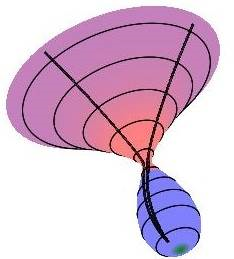Riemannian Geometry

### Stephen Preston

#### Riemannian Geometry and Partial Differential Equations

My research involves viewing the equations of fluid mechanics and similar systems as infinite-dimensional geodesic equations. This allows us to use differential geometry to understand the structure better, for example to study stability of fluid motion (and the predictability of the weather) or to prove theorems about existence of solutions. I also study related physical models like the motion of a whip or a flag on a pole, as well as distance measures between shapes. I can supervise independent study projects for students who are familiar with at least matrix algebra and ordinary differential equations. My research is supported by the Simons Foundation and the National Science Foundation.

### Robert Sibner

#### Analysis, Geometry, and Algebra

I have conducted research (and taught many courses, both at BC and the graduate center) in many areas of mathematics (Analysis, Geometry, and Algebra). My early work was in the field of complex analysis in the area of discontinuous groups and quasiconformal mappings, where I obtained results about symmetries of Riemann surfaces and domains with infinitely many boundary components. Collaborating with my wife, Lesley, a professor of mathematics at NYU (Poly) we began a study of non-linear partial differential equations on Riemannian manifolds. Examples were equations that modeled compressible fluid flow or described minimal surfaces (surfaces of minimal area). While at the Institute for Advanced Study, we incorporated analytic techniques (functional analysis and PDE) to prove some theorems in algebraic geometry. We later considered problems at the intersection of physics and differential geometry, obtaining results in Yang-Mills theory.

A recent interest has been in the intersection of complex analysis and number theory. Since the seventeenth century, numerous proofs have been given of Fermat’s theorem that primes of the form p=4n+1 can be represents as a sum of squares p=x^2+y^2. Using complex analysis techniques, I have recently obtained a new simple and elegant proof of this result. On the other hand, I have answered a question of Maskit’s in complex analysis by giving number theoretic criteria.

My interests span a broad spectrum of classical mathematics and I am open to suggestions to help with independent study in a broad variety of topics.

### Jeff Suzuki

#### History of Mathematics and Mathematics Education

I study the history of mathematics, mathematics education, and the use of mathematics in everyday life. My recent work has included examining the use of mathematics in patent and constitutional law, best-use practices for inquiry-based learning materials in midlevel mathematics courses, and developing Open Educational Resources for lower college mathematics courses.